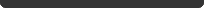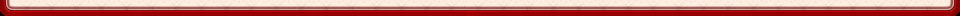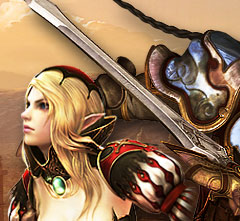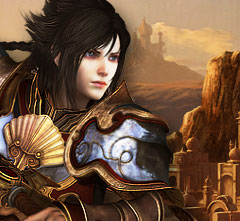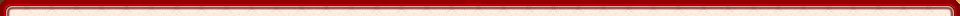远程系（1） 远程系（2） 远程系（3） 法师系（1） 法师系（2） 法师系（3） 近战系（1） 近战系（2）
 远程系主角专用 - 混沌之风 　　0 转威力: 25 + (技能等级-1) * 1 (Lv20=44，Lv40=64，Lv60=84) 　　1 转威力: 50 + (技能等级-1) * 1 (Lv20=69，Lv40=89，Lv60=109) 　　2 转威力: 75 + (技能等级-1) * 1 (Lv20=94，Lv40=114，Lv60=134) 　　3 转威力: 100 + (技能等级-1) * 2 (Lv20=138，Lv40=178，Lv60=218) 　　4 转威力: 125 + (技能等级-1) * 3 (Lv20=182，Lv40=242，Lv60=302) 　　说明 　　消耗行动力150，4 回合内使敌人中毒并持续掉血，威力比巫女的【暗黑的气运】低，没有降低生命力的效果 　　加点建议 　　毒的效果比较一般，但由于可以与近战系主角的【大地的鼓动】组合成新的技能效果，建议加到 40 级左右 　　火枪手 - 乱射 　　0 转威力: 75 + (技能等级-1) * 6 (Lv20=189，Lv40=309，Lv60=429) 　　1 转威力: 200 + (技能等级-1) * 7 (Lv20=333，Lv40=473，Lv60=613)远程篇 (一) 　　2 转威力: 275 + (技能等级-1) * 8 (Lv20=427，Lv40=587，Lv60=747) 　　3 转威力: 350 + (技能等级-1) * 8 (Lv20=502，Lv40=662，Lv60=822) 　　4 转威力: 350 + (技能等级-1) * 13 (Lv20=597，Lv40=857，Lv60=1117) 　　说明 　　消耗行动力150，威力不错。0 转和 1 转可以攻击竖排两个敌人，2 转开始可以攻击竖排三个敌人。 　　技能威力对第一排造成 100% 伤害，对第二排造成 50% 伤害，对第三排造成 25% 伤害。 　　加点建议 　　无论佣兵火枪还是主角火枪都建议点满此技能 　　发明家 - 召唤机器 　　说明 　　消耗行动力 165，根据发明家装备的道具不同，可以召唤出不同的机器，技能本身没有伤害 　　加点建议 　　发明家只有这个技能，点满就行 　　耶尼切里 - 狙击姿势 　　0 转增加攻击力: 10 + (技能等级-1) * 5 (Lv20=105，Lv40=205，Lv60=305) 　　1 转增加攻击力: 20 + (技能等级-1) * 6 (Lv20=134，Lv40=254，Lv60=374) 　　2 转增加攻击力: 30 + (技能等级-1) * 7 (Lv20=163，Lv40=303，Lv60=443) 　　3 转增加攻击力: 40 + (技能等级-1) * 9 (Lv20=211，Lv40=391，Lv60=571) 　　4 转增加攻击力: 50 + (技能等级-1) * 12 (Lv20=278，Lv40=518，Lv60=758) 　　说明 　　不消耗行动力，使用后下一回合攻击力提升 　　加点建议 　　耶尼切里只有这个技能，点满就行 　　火枪系加点建议 　　主角火枪：乱射60，混沌之风40 　　佣兵火枪：乱射60，浑身一击40 　　发 明 家：召唤机器60 　　耶尼切里：狙击姿势60游戏介绍 游戏简介 游戏背景 游戏配置 游戏操作 城市系统 社交系统 道具系统 角色佣兵 角色信息 菜单介绍 物品栏目 微观地图 个人商店 拍卖场所 幸运轮盘 主角职业 剑士 枪手 斧手 火枪手 弓手 炮手 巫师 狂战士 音乐家 佣兵介绍 D级：剑兵 长枪兵 维京海盗 弓兵 火枪兵 炮兵 巫女 僧侣 C级：驯兽师 降魔师 女骑士 预言家 发明家 巨炮兵 魔女 公主 女神官 耶尼切里 B级：斯巴达勇士 NPC资料 任务NPC 道具商人 制作师 技能分析 远程篇(1) 法师篇(1) 近战篇(1) 远程篇(2) 法师篇(2) 近战篇(2)远程篇(3) 法师篇(3) 武器 剑 枪 斧 弓 炮 杖 火枪 乐器 装备道具 远程系套装 魔法系套装 戒 指 近战系套装 咒术书 药水 技能书 地下城迷宫 精灵之森　黄河遗址　克里特迷宫 吴哥窟 摩亨佐达罗墓地　布朗城 君士坦丁堡激战地 泰姬陵的深渊 恶灵丛林　巴比伦空中花园 龙宫 死亡将军城 失落火山谷 昆虫巢穴 宇宙树城　王室峡谷　沉没机械殿 世界地图 全图 东南亚 东北亚 西南亚 中原 欧洲南部 欧洲北部 北美